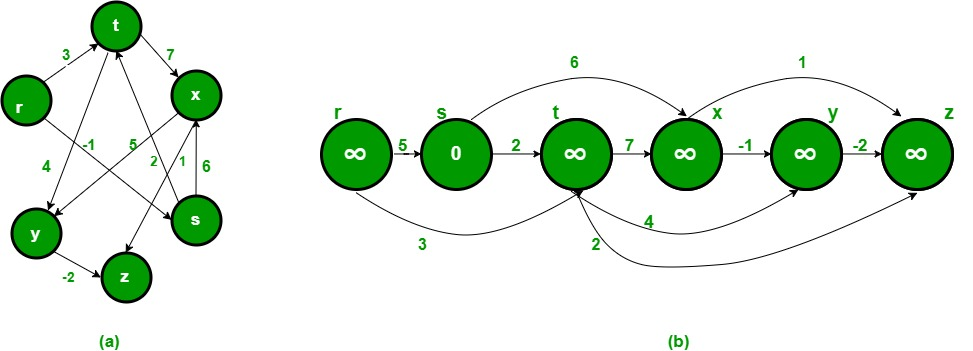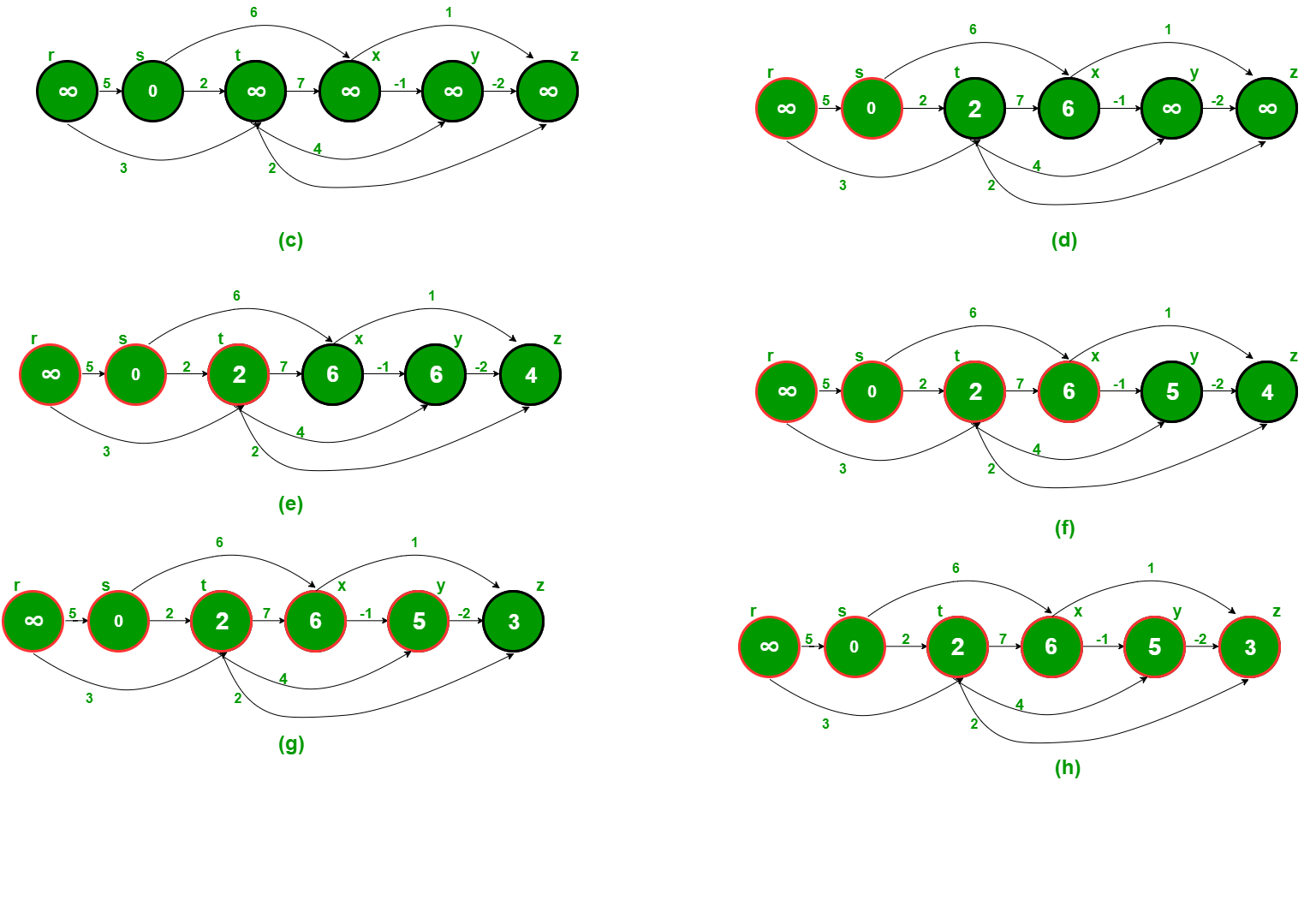# Shortest Path in Directed Acyclic Graph

• Difficulty Level : Medium
• Last Updated : 23 Feb, 2022

Given a Weighted Directed Acyclic Graph and a source vertex in the graph, find the shortest paths from given source to all other vertices.

For a general weighted graph, we can calculate single source shortest distances in O(VE) time using Bellman–Ford Algorithm. For a graph with no negative weights, we can do better and calculate single source shortest distances in O(E + VLogV) time using Dijkstra’s algorithm. Can we do even better for Directed Acyclic Graph (DAG)? We can calculate single source shortest distances in O(V+E) time for DAGs. The idea is to use Topological Sorting.
We initialize distances to all vertices as infinite and distance to source as 0, then we find a topological sorting of the graph. Topological Sorting of a graph represents a linear ordering of the graph (See below, figure (b) is a linear representation of figure (a) ). Once we have topological order (or linear representation), we one by one process all vertices in topological order. For every vertex being processed, we update distances of its adjacent using distance of current vertex.
Following figure is taken from this source. It shows step by step process of finding shortest paths.Following is complete algorithm for finding shortest distances.
1) Initialize dist[] = {INF, INF, ….} and dist[s] = 0 where s is the source vertex.
2) Create a topological order of all vertices.
3) Do following for every vertex u in topological order.
………..Do following for every adjacent vertex v of u
………………if (dist[v] > dist[u] + weight(u, v))
………………………dist[v] = dist[u] + weight(u, v)

## C++

 `// C++ program to find single source shortest paths for Directed Acyclic Graphs``#include``#include ``#define INF INT_MAX``using` `namespace` `std;` `// Graph is represented using adjacency list. Every node of adjacency list``// contains vertex number of the vertex to which edge connects. It also``// contains weight of the edge``class` `AdjListNode``{``    ``int` `v;``    ``int` `weight;``public``:``    ``AdjListNode(``int` `_v, ``int` `_w)  { v = _v;  weight = _w;}``    ``int` `getV()       {  ``return` `v;  }``    ``int` `getWeight()  {  ``return` `weight; }``};` `// Class to represent a graph using adjacency list representation``class` `Graph``{``    ``int` `V;    ``// No. of vertices'` `    ``// Pointer to an array containing adjacency lists``    ``list *adj;` `    ``// A function used by shortestPath``    ``void` `topologicalSortUtil(``int` `v, ``bool` `visited[], stack<``int``> &Stack);``public``:``    ``Graph(``int` `V);   ``// Constructor` `    ``// function to add an edge to graph``    ``void` `addEdge(``int` `u, ``int` `v, ``int` `weight);` `    ``// Finds shortest paths from given source vertex``    ``void` `shortestPath(``int` `s);``};` `Graph::Graph(``int` `V)``{``    ``this``->V = V;``    ``adj = ``new` `list[V];``}` `void` `Graph::addEdge(``int` `u, ``int` `v, ``int` `weight)``{``    ``AdjListNode node(v, weight);``    ``adj[u].push_back(node); ``// Add v to u's list``}` `// A recursive function used by shortestPath. See below link for details``// https://www.geeksforgeeks.org/topological-sorting/``void` `Graph::topologicalSortUtil(``int` `v, ``bool` `visited[], stack<``int``> &Stack)``{``    ``// Mark the current node as visited``    ``visited[v] = ``true``;` `    ``// Recur for all the vertices adjacent to this vertex``    ``list::iterator i;``    ``for` `(i = adj[v].begin(); i != adj[v].end(); ++i)``    ``{``        ``AdjListNode node = *i;``        ``if` `(!visited[node.getV()])``            ``topologicalSortUtil(node.getV(), visited, Stack);``    ``}` `    ``// Push current vertex to stack which stores topological sort``    ``Stack.push(v);``}` `// The function to find shortest paths from given vertex. It uses recursive``// topologicalSortUtil() to get topological sorting of given graph.``void` `Graph::shortestPath(``int` `s)``{``    ``stack<``int``> Stack;``    ``int` `dist[V];` `    ``// Mark all the vertices as not visited``    ``bool` `*visited = ``new` `bool``[V];``    ``for` `(``int` `i = 0; i < V; i++)``        ``visited[i] = ``false``;` `    ``// Call the recursive helper function to store Topological Sort``    ``// starting from all vertices one by one``    ``for` `(``int` `i = 0; i < V; i++)``        ``if` `(visited[i] == ``false``)``            ``topologicalSortUtil(i, visited, Stack);` `    ``// Initialize distances to all vertices as infinite and distance``    ``// to source as 0``    ``for` `(``int` `i = 0; i < V; i++)``        ``dist[i] = INF;``    ``dist[s] = 0;` `    ``// Process vertices in topological order``    ``while` `(Stack.empty() == ``false``)``    ``{``        ``// Get the next vertex from topological order``        ``int` `u = Stack.top();``        ``Stack.pop();` `        ``// Update distances of all adjacent vertices``        ``list::iterator i;``        ``if` `(dist[u] != INF)``        ``{``          ``for` `(i = adj[u].begin(); i != adj[u].end(); ++i)``             ``if` `(dist[i->getV()] > dist[u] + i->getWeight())``                ``dist[i->getV()] = dist[u] + i->getWeight();``        ``}``    ``}` `    ``// Print the calculated shortest distances``    ``for` `(``int` `i = 0; i < V; i++)``        ``(dist[i] == INF)? cout << ``"INF "``: cout << dist[i] << ``" "``;``}` `// Driver program to test above functions``int` `main()``{``    ``// Create a graph given in the above diagram.  Here vertex numbers are``    ``// 0, 1, 2, 3, 4, 5 with following mappings:``    ``// 0=r, 1=s, 2=t, 3=x, 4=y, 5=z``    ``Graph g(6);``    ``g.addEdge(0, 1, 5);``    ``g.addEdge(0, 2, 3);``    ``g.addEdge(1, 3, 6);``    ``g.addEdge(1, 2, 2);``    ``g.addEdge(2, 4, 4);``    ``g.addEdge(2, 5, 2);``    ``g.addEdge(2, 3, 7);``    ``g.addEdge(3, 4, -1);``    ``g.addEdge(4, 5, -2);` `    ``int` `s = 1;``    ``cout << ``"Following are shortest distances from source "` `<< s <<``" n"``;``    ``g.shortestPath(s);` `    ``return` `0;``}`

## Java

 `// Java program to find single source shortest paths in Directed Acyclic Graphs``import` `java.io.*;``import` `java.util.*;` `class` `ShortestPath``{``    ``static` `final` `int` `INF=Integer.MAX_VALUE;``    ``class` `AdjListNode``    ``{``        ``private` `int` `v;``        ``private` `int` `weight;``        ``AdjListNode(``int` `_v, ``int` `_w) { v = _v;  weight = _w; }``        ``int` `getV() { ``return` `v; }``        ``int` `getWeight()  { ``return` `weight; }``    ``}` `    ``// Class to represent graph as an adjacency list of``    ``// nodes of type AdjListNode``    ``class` `Graph``    ``{``        ``private` `int` `V;``        ``private` `LinkedListadj[];``        ``Graph(``int` `v)``        ``{``            ``V=v;``            ``adj = ``new` `LinkedList[V];``            ``for` `(``int` `i=``0``; i();``        ``}``        ``void` `addEdge(``int` `u, ``int` `v, ``int` `weight)``        ``{``            ``AdjListNode node = ``new` `AdjListNode(v,weight);``            ``adj[u].add(node);``// Add v to u's list``        ``}` `        ``// A recursive function used by shortestPath.``        ``// See below link for details``        ``void` `topologicalSortUtil(``int` `v, Boolean visited[], Stack stack)``        ``{``            ``// Mark the current node as visited.``            ``visited[v] = ``true``;``            ``Integer i;` `            ``// Recur for all the vertices adjacent to this vertex``            ``Iterator it = adj[v].iterator();``            ``while` `(it.hasNext())``            ``{``                ``AdjListNode node =it.next();``                ``if` `(!visited[node.getV()])``                    ``topologicalSortUtil(node.getV(), visited, stack);``            ``}``            ``// Push current vertex to stack which stores result``            ``stack.push(``new` `Integer(v));``        ``}` `        ``// The function to find shortest paths from given vertex. It``        ``// uses recursive topologicalSortUtil() to get topological``        ``// sorting of given graph.``        ``void` `shortestPath(``int` `s)``        ``{``            ``Stack stack = ``new` `Stack();``            ``int` `dist[] = ``new` `int``[V];` `            ``// Mark all the vertices as not visited``            ``Boolean visited[] = ``new` `Boolean[V];``            ``for` `(``int` `i = ``0``; i < V; i++)``                ``visited[i] = ``false``;` `            ``// Call the recursive helper function to store Topological``            ``// Sort starting from all vertices one by one``            ``for` `(``int` `i = ``0``; i < V; i++)``                ``if` `(visited[i] == ``false``)``                    ``topologicalSortUtil(i, visited, stack);` `            ``// Initialize distances to all vertices as infinite and``            ``// distance to source as 0``            ``for` `(``int` `i = ``0``; i < V; i++)``                ``dist[i] = INF;``            ``dist[s] = ``0``;` `            ``// Process vertices in topological order``            ``while` `(stack.empty() == ``false``)``            ``{``                ``// Get the next vertex from topological order``                ``int` `u = (``int``)stack.pop();` `                ``// Update distances of all adjacent vertices``                ``Iterator it;``                ``if` `(dist[u] != INF)``                ``{``                    ``it = adj[u].iterator();``                    ``while` `(it.hasNext())``                    ``{``                        ``AdjListNode i= it.next();``                        ``if` `(dist[i.getV()] > dist[u] + i.getWeight())``                            ``dist[i.getV()] = dist[u] + i.getWeight();``                    ``}``                ``}``            ``}` `            ``// Print the calculated shortest distances``            ``for` `(``int` `i = ``0``; i < V; i++)``            ``{``                ``if` `(dist[i] == INF)``                    ``System.out.print( ``"INF "``);``                ``else``                    ``System.out.print( dist[i] + ``" "``);``            ``}``        ``}``    ``}` `    ``// Method to create a new graph instance through an object``    ``// of ShortestPath class.``    ``Graph newGraph(``int` `number)``    ``{``        ``return` `new` `Graph(number);``    ``}` `    ``public` `static` `void` `main(String args[])``    ``{``        ``// Create a graph given in the above diagram.  Here vertex``        ``// numbers are 0, 1, 2, 3, 4, 5 with following mappings:``        ``// 0=r, 1=s, 2=t, 3=x, 4=y, 5=z``        ``ShortestPath t = ``new` `ShortestPath();``        ``Graph g = t.newGraph(``6``);``        ``g.addEdge(``0``, ``1``, ``5``);``        ``g.addEdge(``0``, ``2``, ``3``);``        ``g.addEdge(``1``, ``3``, ``6``);``        ``g.addEdge(``1``, ``2``, ``2``);``        ``g.addEdge(``2``, ``4``, ``4``);``        ``g.addEdge(``2``, ``5``, ``2``);``        ``g.addEdge(``2``, ``3``, ``7``);``        ``g.addEdge(``3``, ``4``, -``1``);``        ``g.addEdge(``4``, ``5``, -``2``);` `        ``int` `s = ``1``;``        ``System.out.println(``"Following are shortest distances "``+``                            ``"from source "` `+ s );``        ``g.shortestPath(s);``    ``}``}``//This code is contributed by Aakash Hasija`

## Python3

 `# Python program to find single source shortest paths``# for Directed Acyclic Graphs Complexity :OV(V+E)``from` `collections ``import` `defaultdict` `# Graph is represented using adjacency list. Every``# node of adjacency list contains vertex number of``# the vertex to which edge connects. It also contains``# weight of the edge``class` `Graph:``    ``def` `__init__(``self``,vertices):` `        ``self``.V ``=` `vertices ``# No. of vertices` `        ``# dictionary containing adjacency List``        ``self``.graph ``=` `defaultdict(``list``)` `    ``# function to add an edge to graph``    ``def` `addEdge(``self``,u,v,w):``        ``self``.graph[u].append((v,w))`  `    ``# A recursive function used by shortestPath``    ``def` `topologicalSortUtil(``self``,v,visited,stack):` `        ``# Mark the current node as visited.``        ``visited[v] ``=` `True` `        ``# Recur for all the vertices adjacent to this vertex``        ``if` `v ``in` `self``.graph.keys():``            ``for` `node,weight ``in` `self``.graph[v]:``                ``if` `visited[node] ``=``=` `False``:``                    ``self``.topologicalSortUtil(node,visited,stack)` `        ``# Push current vertex to stack which stores topological sort``        ``stack.append(v)`  `    ``''' The function to find shortest paths from given vertex.``        ``It uses recursive topologicalSortUtil() to get topological``        ``sorting of given graph.'''``    ``def` `shortestPath(``self``, s):` `        ``# Mark all the vertices as not visited``        ``visited ``=` `[``False``]``*``self``.V``        ``stack ``=``[]` `        ``# Call the recursive helper function to store Topological``        ``# Sort starting from source vertices``        ``for` `i ``in` `range``(``self``.V):``            ``if` `visited[i] ``=``=` `False``:``                ``self``.topologicalSortUtil(s,visited,stack)` `        ``# Initialize distances to all vertices as infinite and``        ``# distance to source as 0``        ``dist ``=` `[``float``(``"Inf"``)] ``*` `(``self``.V)``        ``dist[s] ``=` `0` `        ``# Process vertices in topological order``        ``while` `stack:` `            ``# Get the next vertex from topological order``            ``i ``=` `stack.pop()` `            ``# Update distances of all adjacent vertices``            ``for` `node,weight ``in` `self``.graph[i]:``                ``if` `dist[node] > dist[i] ``+` `weight:``                    ``dist[node] ``=` `dist[i] ``+` `weight` `        ``# Print the calculated shortest distances``        ``for` `i ``in` `range``(``self``.V):``            ``print` `((``"%d"` `%``dist[i]) ``if` `dist[i] !``=` `float``(``"Inf"``) ``else`  `"Inf"` `,end``=``" "``)`  `g ``=` `Graph(``6``)``g.addEdge(``0``, ``1``, ``5``)``g.addEdge(``0``, ``2``, ``3``)``g.addEdge(``1``, ``3``, ``6``)``g.addEdge(``1``, ``2``, ``2``)``g.addEdge(``2``, ``4``, ``4``)``g.addEdge(``2``, ``5``, ``2``)``g.addEdge(``2``, ``3``, ``7``)``g.addEdge(``3``, ``4``, ``-``1``)``g.addEdge(``4``, ``5``, ``-``2``)` `# source = 1``s ``=` `1` `print` `(``"Following are shortest distances from source %d "` `%` `s)``g.shortestPath(s)` `# This code is contributed by Neelam Yadav`

## C#

 `// C# program to find single source shortest``// paths in Directed Acyclic Graphs``using` `System;``using` `System.Collections.Generic;` `public` `class` `ShortestPath``{``    ``static` `readonly` `int` `INF = ``int``.MaxValue;``    ``class` `AdjListNode``    ``{``        ``public` `int` `v;``        ``public` `int` `weight;``        ``public` `AdjListNode(``int` `_v, ``int` `_w) { v = _v; weight = _w; }``        ``public` `int` `getV() { ``return` `v; }``        ``public` `int` `getWeight() { ``return` `weight; }``    ``}` `    ``// Class to represent graph as an adjacency list of``    ``// nodes of type AdjListNode``    ``class` `Graph``    ``{``        ``public` `int` `V;``        ``public` `List[]adj;``        ``public` `Graph(``int` `v)``        ``{``            ``V = v;``            ``adj = ``new` `List[V];``            ``for` `(``int` `i = 0; i < v; ++i)``                ``adj[i] = ``new` `List();``        ``}``        ``public` `void` `addEdge(``int` `u, ``int` `v, ``int` `weight)``        ``{``            ``AdjListNode node = ``new` `AdjListNode(v,weight);``            ``adj[u].Add(node);``// Add v to u's list``        ``}` `        ``// A recursive function used by shortestPath.``        ``// See below link for details``        ``public` `void` `topologicalSortUtil(``int` `v, Boolean []visited,``                                        ``Stack<``int``> stack)``        ``{``            ``// Mark the current node as visited.``            ``visited[v] = ``true``;` `            ``// Recur for all the vertices adjacent to this vertex``            ``foreach``(AdjListNode it ``in` `adj[v])``            ``{``                ``AdjListNode node = it;``                ``if` `(!visited[node.getV()])``                    ``topologicalSortUtil(node.getV(), visited, stack);``            ``}``            ` `            ``// Push current vertex to stack which stores result``            ``stack.Push(v);``        ``}` `        ``// The function to find shortest paths from given vertex. It``        ``// uses recursive topologicalSortUtil() to get topological``        ``// sorting of given graph.``        ``public` `void` `shortestPath(``int` `s)``        ``{``            ``Stack<``int``> stack = ``new` `Stack<``int``>();``            ``int` `[]dist = ``new` `int``[V];` `            ``// Mark all the vertices as not visited``        ``Boolean []visited = ``new` `Boolean[V];``            ``for` `(``int` `i = 0; i < V; i++)``                ``visited[i] = ``false``;` `            ``// Call the recursive helper function to store Topological``            ``// Sort starting from all vertices one by one``            ``for` `(``int` `i = 0; i < V; i++)``                ``if` `(visited[i] == ``false``)``                    ``topologicalSortUtil(i, visited, stack);` `            ``// Initialize distances to all vertices as infinite and``            ``// distance to source as 0``            ``for` `(``int` `i = 0; i < V; i++)``                ``dist[i] = INF;``            ``dist[s] = 0;` `            ``// Process vertices in topological order``            ``while` `(stack.Count != 0)``            ``{``                ``// Get the next vertex from topological order``                ``int` `u = (``int``)stack.Pop();` `                ``// Update distances of all adjacent vertices``                ``if` `(dist[u] != INF)``                ``{``                    ``foreach``(AdjListNode it ``in` `adj[u])``                    ``{``                        ``AdjListNode i= it;``                        ``if` `(dist[i.getV()] > dist[u] + i.getWeight())``                            ``dist[i.getV()] = dist[u] + i.getWeight();``                    ``}``                ``}``            ``}` `            ``// Print the calculated shortest distances``            ``for` `(``int` `i = 0; i < V; i++)``            ``{``                ``if` `(dist[i] == INF)``                    ``Console.Write( ``"INF "``);``                ``else``                    ``Console.Write( dist[i] + ``" "``);``            ``}``        ``}``    ``}` `    ``// Method to create a new graph instance through an object``    ``// of ShortestPath class.``    ``Graph newGraph(``int` `number)``    ``{``        ``return` `new` `Graph(number);``    ``}` `    ``// Driver code``    ``public` `static` `void` `Main(String []args)``    ``{``        ``// Create a graph given in the above diagram. Here vertex``        ``// numbers are 0, 1, 2, 3, 4, 5 with following mappings:``        ``// 0=r, 1=s, 2=t, 3=x, 4=y, 5=z``        ``ShortestPath t = ``new` `ShortestPath();``        ``Graph g = t.newGraph(6);``        ``g.addEdge(0, 1, 5);``        ``g.addEdge(0, 2, 3);``        ``g.addEdge(1, 3, 6);``        ``g.addEdge(1, 2, 2);``        ``g.addEdge(2, 4, 4);``        ``g.addEdge(2, 5, 2);``        ``g.addEdge(2, 3, 7);``        ``g.addEdge(3, 4, -1);``        ``g.addEdge(4, 5, -2);` `        ``int` `s = 1;``        ``Console.WriteLine(``"Following are shortest distances "``+``                            ``"from source "` `+ s );``        ``g.shortestPath(s);``    ``}``}` `// This code is contributed by Rajput-Ji`

Output:

```Following are shortest distances from source 1
INF 0 2 6 5 3 ```

Time Complexity: Time complexity of topological sorting is O(V+E). After finding topological order, the algorithm process all vertices and for every vertex, it runs a loop for all adjacent vertices. Total adjacent vertices in a graph is O(E). So the inner loop runs O(V+E) times. Therefore, overall time complexity of this algorithm is O(V+E).
References:
http://www.utdallas.edu/~sizheng/CS4349.d/l-notes.d/L17.pdf